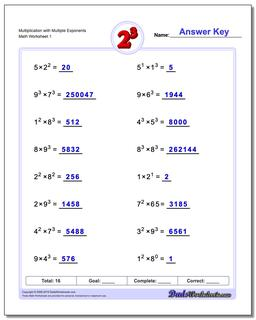# Math Worksheets: Exponents: Exponents: Multiplication with Multiple Exponents## Multiplication with Multiple Exponents

PropertyValue
DescriptionMultiplication with Multiple Exponents: Practice exponents worksheets mixing exponents with simple multiplication. Again, these are fantastic exercises for enhancing student comprehension of basic order of operations concepts where exponents are involved.
Resource TypeWorksheet